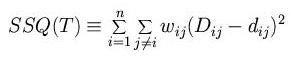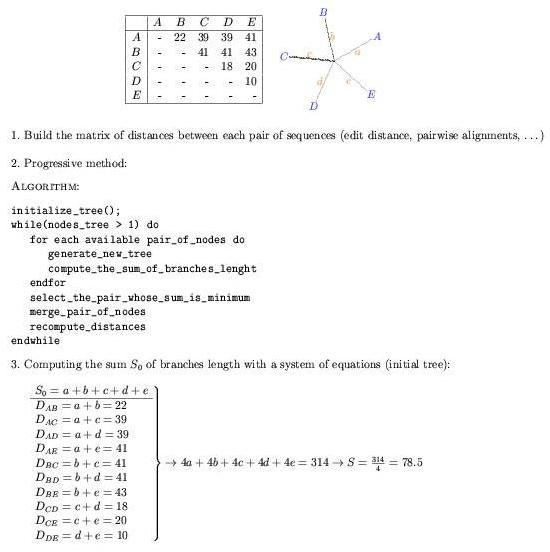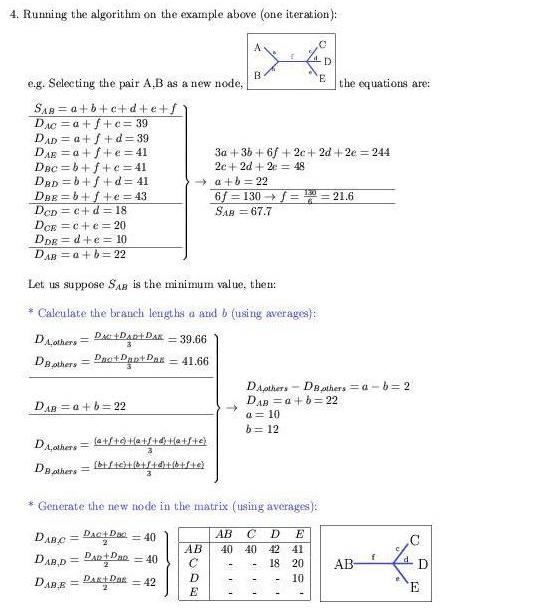9. Neighbour-joining method

 Distance based methods: Minimize the number of global changes between each pair in a group of sequences previously aligned. Least squares approach: From a matrix of distances D between species, find a phylogenetic tree that predicts a set of distances d so that the following expression is minimized:Neighbour-joining: Join the clusters that are close to another and also apart from the rest Heuristic: Minimize the sum of the branch lengths in the tree Additivity of evolutionary distances